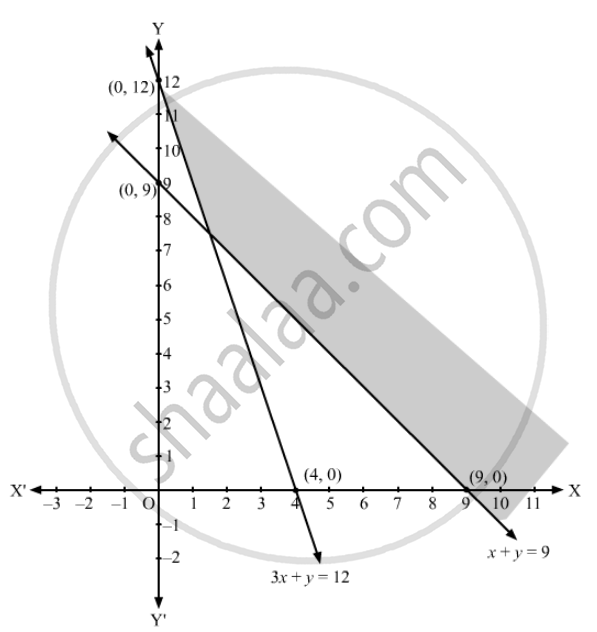Advertisement Remove all ads

# Show that the Solution Set of the Following Linear in Equations is an Unbounded Set: X + Y ≥ 9 3x + Y ≥ 12 X ≥ 0, Y ≥ 0 - Mathematics

Show that the solution set of the following linear in equations is an unbounded set:
x + y ≥ 9
3x + y ≥ 12
x ≥ 0, y ≥ 0

Advertisement Remove all ads

#### Solution

Converting the inequations to equations, we obtain:
x + y = 9, 3x + y = 12 , x = 0, y = 0

y = 9:  This line meets the x-axis at (9, 0) and y-axis at (0, 9). Draw a thick line through these points.
Now, we see that the origin (0, 0) does not satisfy the inequation x + y$\geq$9 Therefore, the potion that does not contain the origin is the solution set to the inequaltion x + y$\geq$9    3x + y = 12:  This line meets the x-axis at (4, 0) and y-axis at (0, 12). Draw a thick line through these points.
Now, we see that the origin (0, 0) does not satisfy the inequation 3x + y$\geq$12. Therefore, the potion that does not contain the origin is the solution set to the inequaltion 3x + y$\geq$

Also, x ≥ 0, y ≥ 0  represents the first quadrant. Hence, the solution set lies in the first quadrant.
We see that in this solution set, the shaded region is unbounded (infinite). Hence, the solution set to the given set of inequalities is an unbounded set.Is there an error in this question or solution?
Advertisement Remove all ads

#### APPEARS IN

RD Sharma Class 11 Mathematics Textbook
Chapter 15 Linear Inequations
Exercise 15.6 | Q 5 | Page 31
Advertisement Remove all ads

#### Video TutorialsVIEW ALL 

Advertisement Remove all ads
Share
Notifications

View all notifications

Forgot password?